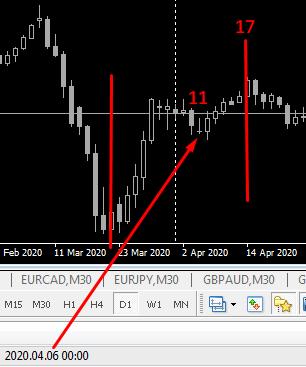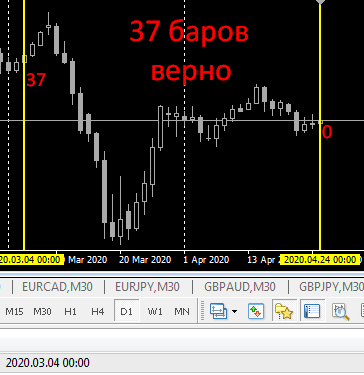# Counting bars including weekends - page 7

Dmitry Fedoseev:

Take the time of one point, use iBarShift() to get the bar number for that time, and then add the required number of bars.

I already did that, didn't help

forex2030:

I've done that before, it didn't work

What was the problem? Look for the error in yourself. In general you are on the right track, there is a mistake somewhere.

forex2030:

I take the top and bottom of a fractal, get the distance between them in pips and draw a line from the last fractal forward for the number of bars equal to those pips
For example, distance = 25 points and from the last fractal, a line is drawn 25 bars ahead

If checked by date, then fractal date + bars = right; if checked by bars, then wrong (less)
As the result, from the date of the fractal, 25 bars are added not 25 calendar days but 25 calendar days and the line rises for example on the 17th bar

and what it draws:

Time[i+(int)Fr]

and without

+ PeriodSeconds()*p_bar

I suspect it will draw a line through the last fractal

Here's taking the point directly from the date 20.03 and adding 17 bars
I got the result on 06.04 which is 11 bars

20 + 17 = 37
31 is the last date of the month
6 new month date
31 + 6 = 37
It turns out that it is not bars on the chart but calendar daysforex2030:

I took the point directly from the date 20.03 and added 17 bars
I got the result 06.04 which is 11 bars

20 + 17 = 37
31 last date of the month
6 new month date
31 + 6 = 37
It turns out that it's not bars on the chart but calendar days

zero bar from right to left is the beginning

Time[i - (int)Fr]

but if you add, then - yes, you calculate not the bars, but the number of days ahead through time, here: ()*p_bar

in this case it is correct - it will be 6.04, i.e. 20+11(March)+6(April)=20March+17days

forex2030:

I do not understand the principle.

The point is that calendar days, not visible bars, are added to the chart

There are 22 bars on the chart, not 30. In a calendar month there are 9 days off, not 16. Why would you lie, since you think they will not check it?

#57

Алексей Тарабанов:

There are 22 bars on the chart, not 30 bars. There are not 16 days off in a calendar month, but about 9. Why would you lie, with the nerve to expect them not to check?

Oops, it's all just about voiced under the fatigue of searching for the truth.

Renat Akhtyamov :

zero bar from right to left is the beginning

maybe you need to subtract rather than add?

Time[i -(int)Fr]

and if you add, then - yes, you are not calculating bars, but the number of days ahead through time

in this case it is correct - it will be 6.04.

Indeed from the zero bar exactly counts bars.
How do you count forward from a point then?forex2030:

Oops, it's all just about voiced under the fatigue of searching for the truth.

Indeed, from a zero bar exactly counts bars.
How then from a point count forward?

well, here it is

Time[i-(int)Fr]

and the loop is like this

for(i=100; i>=0; i--)

with an analysis of at least zero this: i-(int)Fr

but the line to the future(at i<0) all the same - only by time, or add weekend

though.

might suggest - how to shift by a negative number of bars, I can't remember

forex2030:

Oy detective, everything is already roughly voiced under the fatigue of searching for the truth.

It's all been calculated for a long time. The average month is 30.5 days, almost 4.5 weeks. Accordingly, about 22 days of work and almost 9 days off, not counting holidays.

In the interval you highlighted, there are two holidays - Christmas and New Year.

Where do 16 weekends come from and where are the 30 bars? The post is 100% false.

Алексей Тарабанов:

Where did the 16 weekends come from and where are the 30 bars? The post is 100% false.

Get lost, dissolve, disappear, you're useless!!!